# Gaseous and liquid states Questions and Answers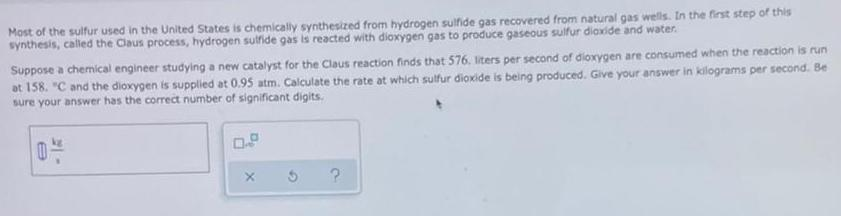Physical Chemistry
Gaseous and liquid states
Most of the sulfur used in the United States is chemically synthesized from hydrogen sulfide gas recovered from natural gas wells. In the first step of this synthesis, called the Claus process, hydrogen sulfide gas is reacted with dioxygen gas to produce gaseous sulfur dioxide and water. Suppose a chemical engineer studying a new catalyst for the Claus reaction finds that 576. liters per second of dioxygen are consumed when the reaction is run at 158. "C and the dioxygen is supplied at 0.95 atm. Calculate the rate at which sulfur dioxide is being produced. Give your answer in kilograms per second. Be sure your answer has the correct number of significant digits. 0- X ?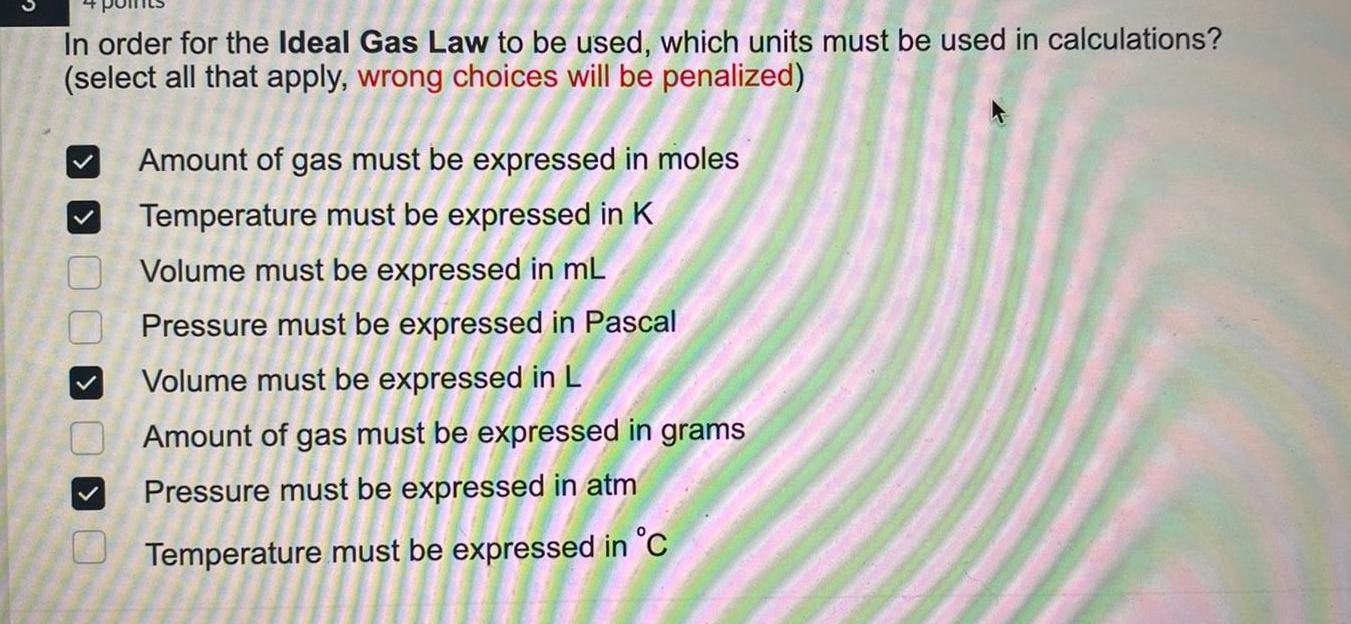Physical Chemistry
Gaseous and liquid states
In order for the Ideal Gas Law to be used, which units must be used in calculations? (select all that apply, wrong choices will be penalized) Amount of gas must be expressed in moles Temperature must be expressed in K Volume must be expressed in mL Pressure must be expressed in Pascal Volume must be expressed in L Amount of gas must be expressed in grams Pressure must be expressed in atm Temperature must be expressed in °C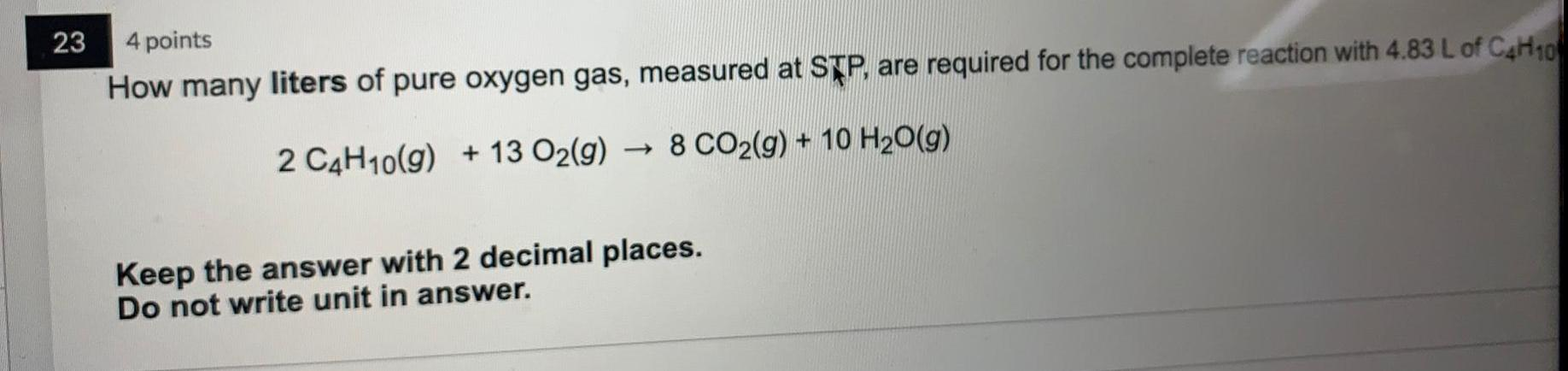Physical Chemistry
Gaseous and liquid states
How many liters of pure oxygen gas, measured at STP, are required for the complete reaction with 4.83 L of C4H10. 2 C4H10(g) + 13 O₂(g) → 8 CO₂(g) + 10 H₂O(g) Keep the answer with 2 decimal places. Do not write unit in answer.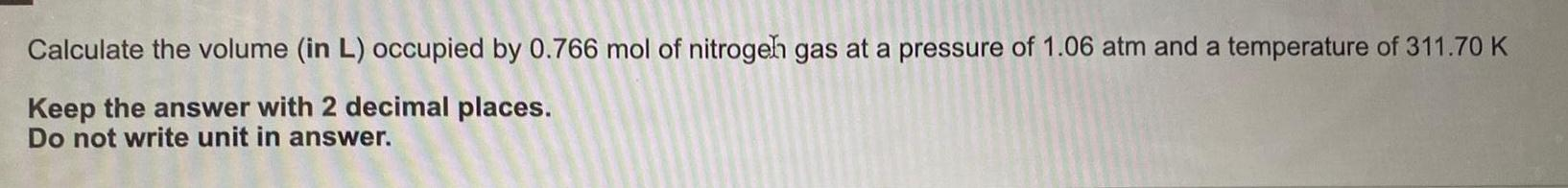Physical Chemistry
Gaseous and liquid states
Calculate the volume (in L) occupied by 0.766 mol of nitrogen gas at a pressure of 1.06 atm and a temperature of 311.70 K Keep the answer with 2 decimal places. Do not write unit in answer.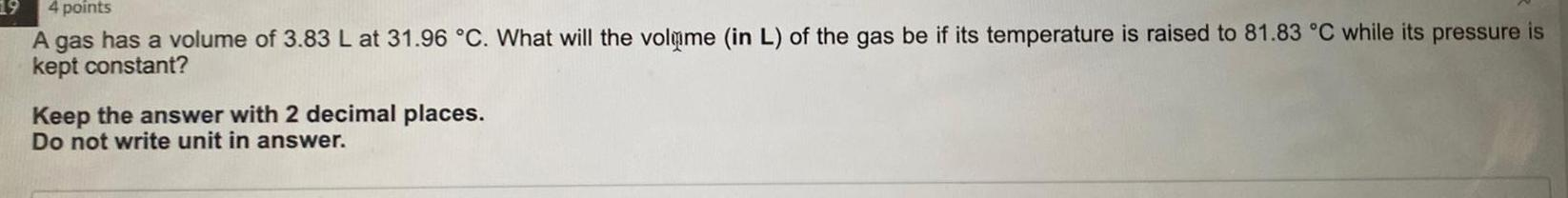Physical Chemistry
Gaseous and liquid states
A gas has a volume of 3.83 L at 31.96 °C. What will the volume (in L) of the gas be if its temperature is raised to 81.83 °C while its pressure is kept constant? Keep the answer with 2 decimal places. Do not write unit in answer.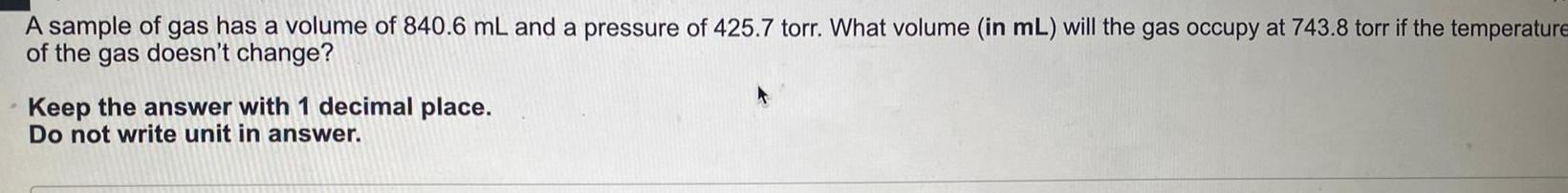Physical Chemistry
Gaseous and liquid states
A sample of gas has a volume of 840.6 mL and a pressure of 425.7 torr. What volume (in mL) will the gas occupy at 743.8 torr if the temperature of the gas doesn't change? Keep the answer with 1 decimal place. Do not write unit in answer.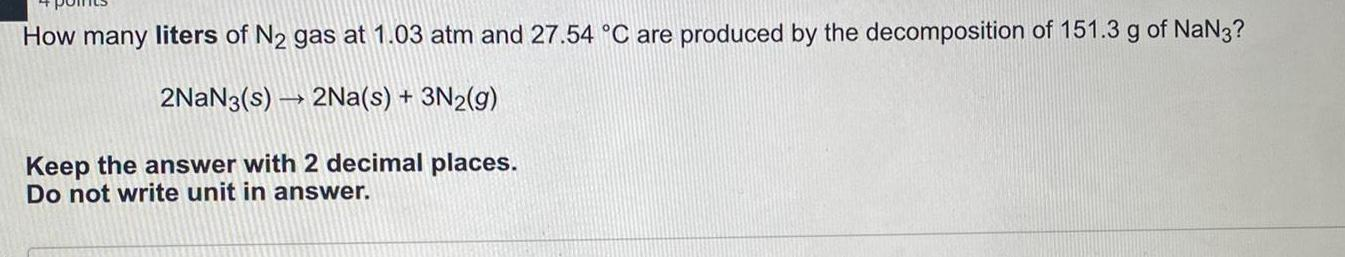Physical Chemistry
Gaseous and liquid states
How many liters of N₂ gas at 1.03 atm and 27.54 °C are produced by the decomposition of 151.3 g of NaN3? 2NaN3(s)→ 2Na(s) + 3N₂(g) Keep the answer with 2 decimal places. Do not write unit in answer.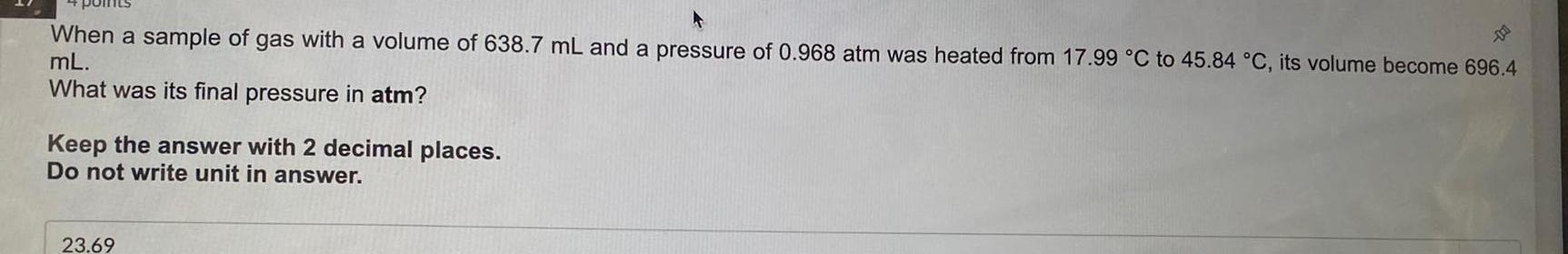Physical Chemistry
Gaseous and liquid states
When a sample of gas with a volume of 638.7 mL and a pressure of 0.968 atm was heated from 17.99 °C to 45.84 °C, its volume become 696.4 mL. What was its final pressure in atm? Keep the answer with 2 decimal places. Do not write unit in answer.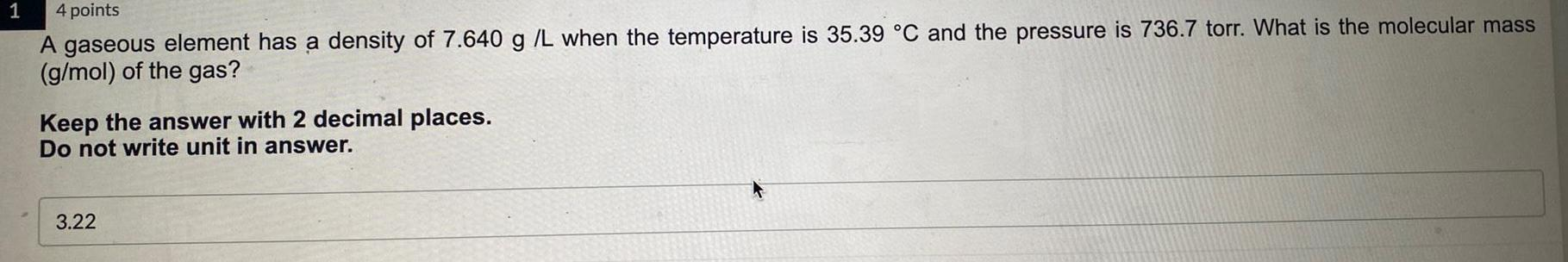Physical Chemistry
Gaseous and liquid states
A gaseous element has a density of 7.640 g /L when the temperature is 35.39 °C and the pressure is 736.7 torr. What is the molecular mass (g/mol) of the gas? Keep the answer with 2 decimal places. Do not write unit in answer. 3.22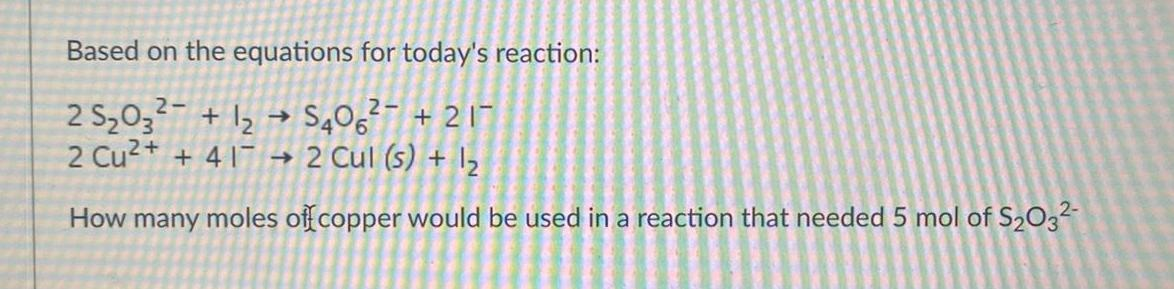Physical Chemistry
Gaseous and liquid states
Based on the equations for today's reaction: 2 S₂O3²- + I2 --> S40O6²- +2I- 2 Cu²+ + 4I- → 2 Cul (s) + I₂ How many moles of copper would be used in a reaction that needed 5 mol of S₂O3²-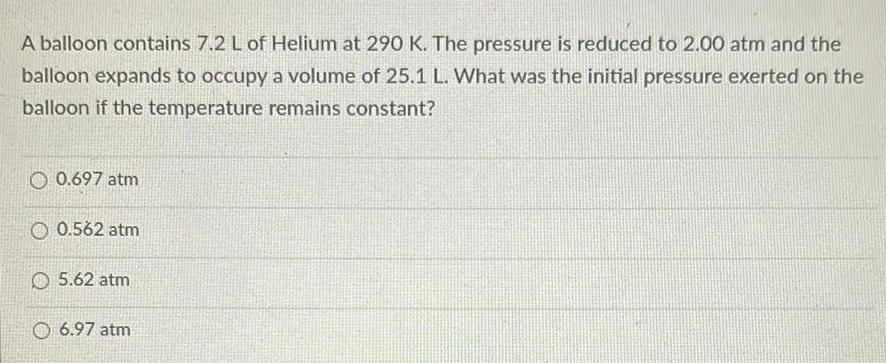Physical Chemistry
Gaseous and liquid states
A balloon contains 7.2 L of Helium at 290 K. The pressure is reduced to 2.00 atm and the balloon expands to occupy a volume of 25.1 L. What was the initial pressure exerted on the balloon if the temperature remains constant? 0.697 atm 0.562 atm 5.62 atm 6.97 atm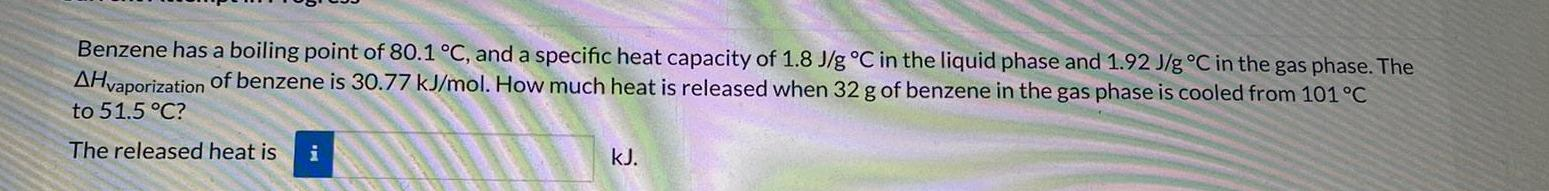Physical Chemistry
Gaseous and liquid states
Benzene has a boiling point of 80.1 °C, and a specific heat capacity of 1.8 J/g °C in the liquid phase and 1.92 J/g °C in the gas phase. The ΔHvaporization of benzene is 30.77 kJ/mol. How much heat is released when 32 g of benzene in the gas phase is cooled from 101 °C to 51.5 °C?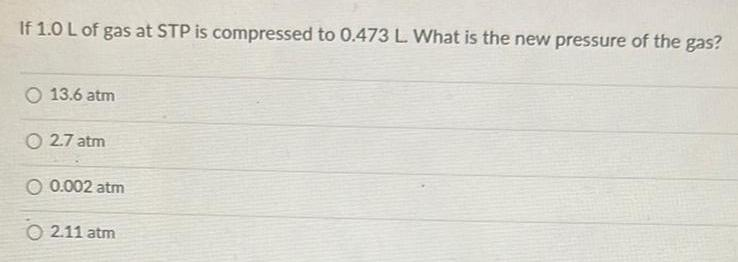Physical Chemistry
Gaseous and liquid states
If 1.0 L of gas at STP is compressed to 0.473 L. What is the new pressure of the gas? 13.6 atm 2.7 atm 0.002 atm 2.11 atm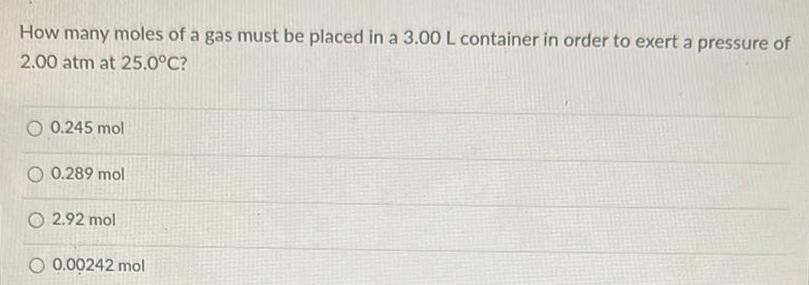Physical Chemistry
Gaseous and liquid states
How many moles of a gas must be placed in a 3.00 L container in order to exert a pressure of 2.00 atm at 25.0°C? 0.245 mol 0.289 mol 2.92 mol 0.00242 mol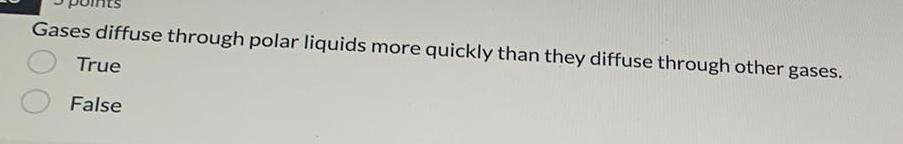Physical Chemistry
Gaseous and liquid states
Gases diffuse through polar liquids more quickly than they diffuse through other gases. True False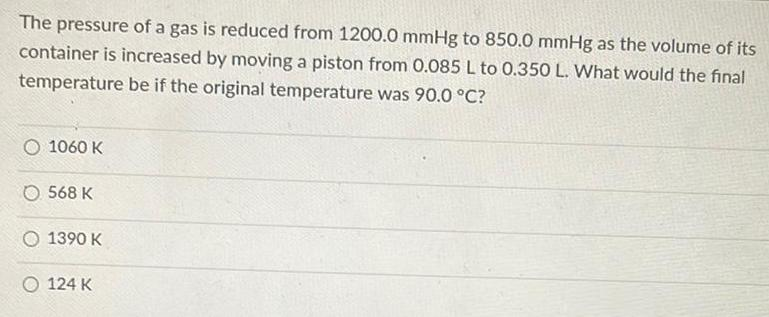Physical Chemistry
Gaseous and liquid states
The pressure of a gas is reduced from 1200.0 mmHg to 850.0 mmHg as the volume of its container is increased by moving a piston from 0.085 L to 0.350 L. What would the final temperature be if the original temperature was 90.0 °C? 1060 K 568 K 1390 K 124 K